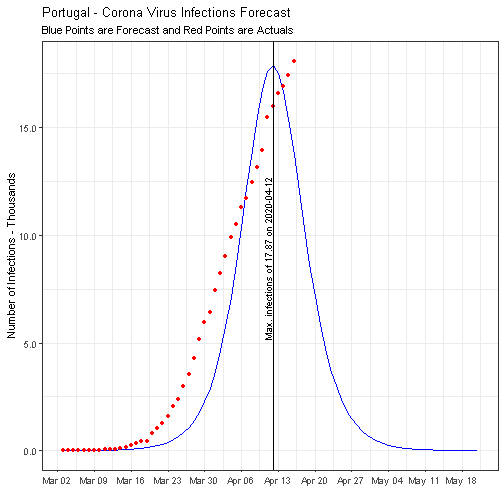# COVID-19 outbreak: the case of Portugal(Update)

### COVID-19: a data epidemic

With the outbreak of COVID-19 one thing that is certain is that never before a virus has gone so much viral on the internet. Especially, a lot of data about the spread of the virus is going around.

In the space of a few weeks, complex terms like “group immunity” (herd immunity) or “flattening the curve” started to circulate on social networks.

Here we will try an approach that demonstrates the limitations of the model from the previous post The importance of “flattening the curve”.
This post is based on Contagiousness of COVID-19 Part I: Improvements of Mathematical Fitting (Guest Post), this post compared to the previous one shows that the fitting of the data with the SIR model is tricky, the general problem is that the fitting-algorithm is not always finding it’s way to the best solution.

### Why doesn’t the algorithm converge to the optimal solution?

There are two main reasons why the model is not converging well.

### Early stopping of the algorithm

The first reason is that the optim algorithm is stopping too early before it has found the right solution.

### Ill-conditioned problem

The second reason for the bad convergence behaviour of the algorithm is that the problem is ill-conditioned.

### The latest data from:

https://github.com/CSSEGISandData/COVID-19/tree/master/csse_covid_19_data/csse_covid_19_time_series

### Currently the calculation of R0 by the model of the previous post

gives: R0 = 1.2777406

### R0 by the current model

The current model gives: R0 = 1.0620105 as we see the result is quite different.

### Some differences of the SIR model

This SIR model uses the parameters $K = \beta - \gamma$ and $R_0 = \frac{\beta}{\gamma}$### Final conclusions

Based on this model the peak of the epidemic has already happened on day 2020-04-12 with a maximum of 17 870.
This is all we want, but it doesn’t mean it is correct. Just because we use a mathematical model does not mean that our conclusions/predictions are trustworthy. We need to challenge the premises which are the underlying data and models.

If you liked this post, you can share it with your followers or follow me on Twitter!